`mailto:` blog -at- heyrick -dot- eu

## Some end of year geekery: AsmToHTML - marking up assembler source to HTML

Since my time spent on the ROOL forums now is... minimal... this gives me extra time to do other things. Like Mamie's level editor. Or throwing together, well, this...

For my Mamie articles, one part was an assembler version of the original code to make the flash effect work. It took "effing ages" to mark up that code, by hand.

So I thought I'd make a little program to do it for me.

Now, the layout of assembler (ObjAsm format) is pretty simple. Anything flush with the left is some sort of label.
If it is indented, it's an opcode. I use the word opcode rather than instruction, as this also counts for pseudo-instructions like `ADR` and whatever may have been defined as macros.
Following that, it's some sort of parameter. A register, a label, and address...

The colourisation is based upon that provided by Zap, so numbers are coloured in one way and things like brackets, hash characters, etc differently.

In keeping with the layout of my blog, the colouring is directly inserted into the file. However it uses a set of strings so this could be altered to refer to external CSS without too much bother. You have the source, feel free to tweak.

### Licence

This code is licenced under the CDDL. A copy is provided, or you can read it here.

Note also that if you make changes and modifications, it's a kindness to feed them back to me so that I can incorporate them into the available code, and everybody benefits.
I say this, as I've had comments and emails regarding my NetRadio and people adding various extensions and enhancements. Did I see any actual code? Not a bit. It's nice that people are benefitting from my work, but it would be nicer yet if they also shared...

The program begins thus:

```/* AsmToHTML version 0.01

Tuesday, 28th December 2021

Licenced under the CDDL.

NOTE: Our standard behaviour is to bail
the moment a problem is encountered.

*/

#include <stdio.h>
#include <stdlib.h>
#include <string.h>
#include <ctype.h>
#ifdef __riscos
#include "kernel.h"
#include "swis.h"
#endif

#define UNDEFINED 0
#define LABEL     1
#define OPCODE    2
#define COMMENT   3
#define STRING    4
#define NUMBER    5
#define BRACKET   6
#define PARAM     7
#define OPERATOR  8

#define TRUE      1
#define FALSE     0
#define NEWLINE   10

static char *code = NULL;      // Where the code is loaded
static char *markup = NULL;    // How to mark this code up
static unsigned long size = 0; // Size of input file

// We use directly inlined markup to save requiring style sheets and such.
// Feel free to edit this as appropriate.

static char preamble[]      = "<pre style=\"background: black; color: white; overflow-x: auto;\"><b>";
static char postamble[]     = "</b></pre>\n<p>\n\n";

// Colours to match Zap (more or less)
static char label_start[]   = "<font color=\"lemonchiffon\">";
static char opcode_start[]  = "<font color=\"yellow\">";
static char comment_start[] = "<font color=\"lime\"><i>";
static char string_start[]  = "<font color=\"cyan\">";
static char number_start[]  = "<font color=\"orange\">";
static char bracket_start[] = "<font color=\"white\">";
static char param_start[]   = "<font color=\"lemonchiffon\">";
static char operator_start[]= "<font color=\"lightsalmon\">";
static char comment_end[]   = "</i></font>";
static char all_end[]       = "</font>";

static void markup_file(void);
static void output_file(int cnt, char *filename);```

It is worth noting that there are two primary arrays. The first, `code`, is where the assembler source is loaded.
The second, `markup`, is the exact same size as code, and it carries a value representing how that character is supposed to be coloured.

### The main() function

The main function is pretty simple.

```int main(int argc, char *argv[])
{
if ( ( argc < 2 ) && ( argc > 3 ) )
{
printf("Syntax: AsmToHTML <input> [<output>]\n");
exit(EXIT_FAILURE);
}

markup_file();

output_file(argc, argv);

exit(EXIT_SUCCESS);
}```

There are two ways in which to use AsmToHTML:

• `AsmToHTML inputfile outputfile`
Perhaps the most useful, this will read the given input file and write the marked up version to the specified output file.
• `AsmToHTML inputfile`
This way has no output file, so output is written to the screen. This may be useful if. say. one is running AsmToHTML in a TaskWindow, as you can simply select the markup and paste it directly into your document.

```static void load_file(char *filename)
{
// Loads the specified file and sets up memory for starting.

FILE *fp = NULL;
unsigned long posn = 0;
unsigned int  byte = 0;

// Open the file
fp = fopen(filename, "rb");
if ( fp == NULL )
{
printf("Unable to open input file \"%s\".\n", filename);
exit(EXIT_FAILURE);
}

// Work out how big the file is
fseek(fp, 0, SEEK_END);
size = ftell(fp);
fseek(fp, 0, SEEK_SET);

// Allocate memory
code = malloc((unsigned int)size);
if ( code == NULL )
{
printf("Unable to allocate %lu bytes to load source file.\n", size);
exit(EXIT_FAILURE);
}

markup = malloc((unsigned int)size);
if ( markup == NULL )
{
printf("Unable to allocate %lu bytes for markup.\n", size);
exit(EXIT_FAILURE);
}

for (posn = 0; posn < size; posn++)
{
byte = fgetc(fp);
code[posn] = byte;
markup[posn] = UNDEFINED;

// Fault control codes
if ( byte < 31 )
{
// ...except Tab and newlines ;-)
if ( ( byte != 9 ) && ( byte != NEWLINE ) )
{
printf("Unexpected character (&%02X) in source (at +%lu), aborting.\n", byte, posn);
exit(EXIT_FAILURE);
}
}
}

// Close the file
fclose(fp);

// Done.
return;
}```

The reason we go to all of this palavar, rather than simply using OS_GBPB or OS_File to block-load it directly to memory is so that it will work on other systems. Granted, it's probably not as useful to mark up ObjAsm format assembler when using DOS or Windows, but hey, the option exists.
Additionally, whilst we lose a small amount of speed loading in the file byte by byte, we do gain the ability to reject files with weird and unexpected characters before we end up choking on them.

There's no specific support for characters over 127. It might be worth adding some code to output something like `&#xxx;` for them? When I was checking for unexpected characters, I was primarily thinking of trying to mark up binaries by mistake.

### Byte checking functions

This first function will check if the given byte is whitespace. It differs from the standard isblank() function in the ctype header by also considering a linefeed to be a valid whitespace character.
As it turns out, this was not necessary as isblank also checks for '\r', '\n', '\v' and '\f'; however the header description implied it was only space and tab saying exactly:
`/* non-0 iff c is a blank char: ' ', '\t'. */`
and I couldn't be bothered to fix the program once I found out that the header was economic with the truth...

```static int  iswhite(int b)
{
// Return TRUE if whitespace
// Differs from ifblank() in that we also count a newline as whitespace

if ( ( b == 9 ) || ( b == NEWLINE ) || ( b == 32 ) )
return TRUE;

return FALSE;
}```

This next function will look for what is a valid character next to a number. Brackets, maths symbols, and so on. This will make sense later on.

```static int  isnumsep(int b)
{
// Return TRUE if this is a character that could be around a number

char numsep[] = "{}[]():+-*/<>. =&_,\x0A";

if ( strchr(numsep, b) == NULL )

return TRUE; // b was found
}```

### Marking up the code

Okay, here's the hard part. I'll break in and out of the function as explanations are required.

```static void markup_file(void)
{
// Process through the file adjusting the markup block according to what we find.

unsigned long posn = 0;      // offset to byte being processed
int  byte = 0;               // value of byte being processed

int  inlabel = TRUE;         // are we in a label?
int  seenopcode = FALSE;     // have we seen the opcode part yet?
int  inopcode = FALSE;       // are we in the opcode?
int  incomment = FALSE;      // are we in a comment?
int  instring = FALSE;       // are we in a string?
int  innumber = FALSE;       // are we in a denary number?
int  inhexnum = FALSE;       // are we in a hex number?
int  inopera = FALSE;        // are we in an operator?

while ( posn < size )
{
byte = code[posn];

// Sequence handling
// =================
```

Note that "inlabel" starts off as TRUE. Anything to the far left of the line is considered a label.

Comments are handled by simply swallowing characters until the newline is reached.

```      if ( incomment )
{
// Handle comments - ignore everything until the end of the line.
if ( byte == NEWLINE )
{
incomment = FALSE;
}
else
{
markup[posn] = COMMENT;

// back to the for loop, we're done here
posn++;
continue;
}
}```

String handling continues until we reach a closing quote. ObjAsm has an option to support the use of C style string modifiers such as `\t` and `\n`. The ObjAsm user manual does not specify exactly which ones are supported, so I'm assuming all by simply swallowing the following byte. This means that "`This is a \"string\".`" works (something Zap gets wrong ☺).

As I'm writing this, I'm wondering if ObjAsm supports C-like single character strings (of the form `'x'`). If so, this really ought to be added, but added as a seperate bit of code so that only quotes can close a quotes string, and only apostrophe can close an apostrophe string.

```      // Are we in a string?
if ( instring )
{
markup[posn] = STRING;

if ( byte == '\\' )
{
// It's a "\X" sequence, so swallow a character
// in order that "this is a \"string\" works
// correctly.
posn++;
markup[posn] = STRING;
}

if ( byte == '\"' )
{
// End of string
instring = FALSE;
}

// back to the for loop, we're done here
posn++;
continue;
}```

We begin in a label. This state is cancelled by either finding a semi-colon (comment marker) or by any character that is whitespace.

```      // Are we in a label?
if ( inlabel )
{
// Special handling for comment beginning a line
if ( byte == ';' )
{
inlabel = FALSE;
incomment = TRUE;
markup[posn] = COMMENT;

// back to the for loop, we're done here
posn++;
continue;
}

if ( iswhite(byte) )
{
// We've reached whitespace - it's the end of the label
inlabel = FALSE;
markup[posn] = UNDEFINED;
}
else
{
// It still the label
markup[posn] = LABEL;

// back to the for loop, we're done here
posn++;
continue;
}
}```

In order to support operators (`:BASE:`, `:NOT:`, `:SHL:`, etc), we detect an opening colon, and here we continue until there's a closing colon.

```      if ( inopera )
{
// Handle operators
if ( byte == ':' )
{
inopera = FALSE;
markup[posn] = OPERATOR;
}
else
{
markup[posn] = OPERATOR;
}

// back to the for loop, we're done here
posn++;
continue;
}```

Once we have determined that what follows is a number, we continue processing as a number so long as it's a valid digit (including with decimal point) or an underscore (for `2_10101` style notation). Note that hex numbers are handled below.

```      // Are we in a number?
if ( innumber )
{
if ( isdigit(byte) || ( byte == '_' ) || ( byte == '.' ) )
{
// It's part of the number
markup[posn] = NUMBER;
posn++;
continue; // back to the for loop, we're done here.
}
else
{
// It's not a number, a dot, or an underscore so end the number
innumber = FALSE;
markup[posn] = UNDEFINED;
}
}```

We're not done with numbers yet. There's another form of notation that is very common, and that is hexadecimal, or base 16. These numbers use 0 to 9 as normal, and then extend through A to F; with F meaning '15', which means 10 in hex is the same as 16 in denary.
These are handled seperately in order that we know that a number is in hex, rather than assuming it as an option for all numbers and getting in a muddle if we see something like "DEFINE". Is that DEF as a number, or what?

```      // Are we in a hex number?
if ( inhexnum )
{
if ( isxdigit(byte) )
{
// It's part of the number
markup[posn] = NUMBER;
posn++;
continue; // back to the for loop, we're done here.
}
else
{
// It's not A-F (a-f), or 0-9 end the number
inhexnum = FALSE;
markup[posn] = UNDEFINED;
}
}```
There are no extra characters here, as "A4F.3E" is not a valid number. Hex only works for whole numbers. And while "16_DEADBABE" might be valid (I don't know), it's an extremely bizarre way to write it.

Now we can begin with handling bytes rather than states.
We begin with handling whitespace, and as a side effect if we were processing an opcode, marking the end of the opcode.

```      // Regular handling
// ================

if ( iswhite(byte) )
{
// Whitespace
markup[posn] = UNDEFINED;

// If we were in an opcode, we are no longer
if ( inopcode == TRUE )
inopcode = FALSE;
}```

And if it wasn't whitespace? Well, if we're in a label we should mark it as such. I'm not sure this code is actually used. The label handling above ought to deal with this. This part is slightly older code that could possibly be removed.

```      else
{
// NOT whitespace
if ( inlabel )
{
// It's the label
markup[posn] = LABEL;
}```

If it's not the label, then it's either the opcode or the parameter. How we tell the two apart is to check the "seenopcode" flag. If we haven't yet seen the opcode, then this must be it.

```         else
{
// It's not the label, so must be opcode or parameter
if ( seenopcode == FALSE )
{
// Opcode not yet seen, so this must be an opcode
seenopcode = TRUE;
inopcode = TRUE;
markup[posn] = OPCODE;
}```

On the other hand, if we have seen the opcode, either this is it or it's part of the parameter.

```            else
{
if ( inopcode )
{
// Still dealing with the opcode?
markup[posn] = OPCODE;
}
else
{
// Not opcode, so must be a parameter
markup[posn] = PARAM;
}
}
}```

Now we've worked out whether it's a label, opcode, or parameter, or whitespace, the colour will have been set accordingly.
Now it's time to override that according to special cases. The first of which is picking up on a semi-colon to introduce comments.

```         // Now pluck up some special behaviours

if ( byte == ';' )
{
// It's the start of a comment
incomment = TRUE;
markup[posn] = COMMENT;
}```

The second check is for operators. If we encounter a ':' then this is the case.

```         if ( byte == ':' )
{
// It's the start of an operator
inopera = TRUE;
markup[posn] = OPERATOR;
}```

Next, check to see if it's quotes to open a string.

```         if ( byte == '\"' )
{
// It's a quote, so switch to "string" mode
instring = TRUE;
markup[posn] = STRING;
}```

Now, look to see if it's some sort of brackets. Zap also colours hash, circumflex, and comma in white, so we'll include these too. There may be some other characters which may need to be added?
Then, because square brackets introducing conditional compilation are coloured differently, we shall pluck these out and colour them correctly.

```         // Handle brackets and stuff
if ( ( byte == '{' ) || ( byte == '}' ) ||
( byte == '[' ) || ( byte == ']' ) ||
( byte == '<' ) || ( byte == '>' ) ||
( byte == '(' ) || ( byte == ')' ) ||
( byte == '#' ) || ( byte == '^' ) ||
( byte == '@' ) || ( byte == ',' ) )
{
// {}, [], <>, (), #, ^, @, and ,
markup[posn] = BRACKET;

// Special handling for conditional compilation
if ( inopcode && ( ( byte == '[' ) || ( byte == ']' ) ) )
markup[posn] = OPCODE;
}```

If the byte is a hash, then what follows ought to be a number.

```         // Special handling for '#', what follows is a number
// but we leave the '#' as white
if ( byte == '#' )
{
innumber = TRUE; // don't add markup, leave it as BRACKET (white)
}```

We don't need to code in any support for telling the difference between a base ten number and a hex number (like `#&FF`) because we can make use of a cheat. If this is the case, the '#' begins a number. The number processing will see the following '&' and determine that it isn't a number, at which point it'll trickle through the code until this next part where it will be picked up as a hex number.

Thus, what follows an ampersand is a hex number. By only accepting hex numbers beginning with '&', we can remedy a lot of weird pathological cases in the parser. So let's introduce a hex number now.

```         // Special handling for '&', what follows is a hex number
// and we consider the '&' to be a part of that.
if ( byte == '&' )
{
inhexnum = TRUE;
markup[posn] = NUMBER;
}```

How handle finding a number on its own. This may happen with things like "`, 10, 0`" following a string.
The check to ensure we've seen the opcode, and we aren't in the opcode, is in order that we know we're looking at where a number should be.

What we do here, to try to reduce the possibility of bogus interpretation, is to look at the byte before the number to ensure that it is a valid numerical seperator; and then we look ahead to ensure the number ends with a valid numerical seperator.
In this way, `:8:` will have the '8' seen as a number, but `the8ball` will not.

```         if ( isdigit(byte) && seenopcode && !inopcode )
{
// We have come across a digit, is this something that we can count as a number?
if ( isnumsep(code[posn-1]) )
{
// Okay, what comes before could make this a number, so
// now look ahead to ensure that this is really a number.

unsigned long offs = posn;
int isnum = TRUE;
int endscan = FALSE;
int chk = 0;

while ( isnum && !endscan )
{
chk = code[offs];

if ( !isdigit(chk) )
{
// No longer a number, so is this a valid number separator?

if ( !isnumsep(chk) )
{
// No, it isn't. So this probably isn't a number
isnum = FALSE;
}

endscan = TRUE; // end of number found, so stop looking
}
offs++;
}

if ( isnum )
{
// Looks like a number to us...
innumber = TRUE;
markup[posn] = NUMBER;
}
}
}```

Zap colourises shift specifiers in an instruction as opcodes. That is to say, `ADDCC PC, PC, R11, LSL#2` will have the ADDCC and LSL both coloured yellow.
So we do the same thing.

If we aren't in the opcode (and we know we can't be in the label or a string or a comment), then we look to see if the previous byte is whitespace or a comma, and if it is, then is the current byte A, L, or R?
If it is, then extract the current byte and the following two in order to see if they are one of LSL, LSR, ASL, ROR, or RRX. If they are, then colour them as opcodes and advance the pointer over them.

```         // Special handling for LSL, LSR, ASR, ROR, and RRX.
if ( inopcode == FALSE )
{
// Not in an opcode, so must be a parameter,
// therefore, do we have a shift specifier?

// MUST follow a comma or whitespace, else we'll end
// up highlighting the "ror" in "error"!
if ( iswhite(code[posn-1]) || ( code[posn-1] == ',' ) )
{
// Is it 'A', 'L', or 'R'?
if ( ( byte == 'A' ) || ( byte == 'a' ) ||
( byte == 'L' ) || ( byte == 'l' ) ||
( byte == 'R' ) || ( byte == 'r' ) )
{
// First byte was 'A', 'L' or 'R', so let's work out the rest
// using a specific string match for only valid combinations.

char oplook;

oplook = tolower(byte);
oplook = tolower( code[posn+1] );
oplook = tolower( code[posn+2] );
oplook = '\0';

if ( ( strcmp(oplook, "lsl") == 0 ) ||
( strcmp(oplook, "lsr") == 0 ) ||
( strcmp(oplook, "asr") == 0 ) ||
( strcmp(oplook, "ror") == 0 ) ||
( strcmp(oplook, "rrx") == 0 ) )
{
markup[posn++] = OPCODE;
markup[posn++] = OPCODE;
markup[posn]   = OPCODE;
}
}
}
}

} // end of "is not whitespace" block```

The final thing to do is the newline handling. This resets everything to the state that it should be for the beginning of a line.

```      // Newline handling
if ( byte == NEWLINE )
{
markup[posn] = UNDEFINED;
inlabel = TRUE;         // reset this for new line
seenopcode = FALSE;
inopcode = FALSE;
incomment = FALSE;
instring = FALSE;
innumber = FALSE;
inhexnum = FALSE;
inopera = FALSE;
}```

And, of course, increment the pointer for the next character.

```      // Next byte
posn++;
}

return;
}```

### Markup output

The output isn't as complicated as it looks. At the start, we either open a file or set the file handle to stdout (so it gets written to the screen).
Then we simply whip through the code outputting it with markup. However we keep track of the "previous" markup value, so that we only change style when necessary. It's a simple but blindlingly obvious optimisation.
When that is done, we tidy up and - if using RISC OS - set the output file's type to &FAF (HTML).

```static void output_file(int cnt, char *filename)
{
FILE *fp = NULL;
unsigned long posn;
int  prevmarkup = UNDEFINED;
int  first = TRUE;

// Handle the output behaviour
if ( cnt == 3 )
{
// Open a file for output
fp = fopen(filename, "wb");
if ( fp == NULL )
{
printf("Unable to open output file \"%s\".\n", filename);
exit(EXIT_FAILURE);
}
}
else
{
// No file specified, so just output to console
fp = stdout;
}

// Do the output

// Write the HTML to begin the code block
fprintf(fp, preamble);

// Process the marked up data
for (posn = 0; posn < size; posn++)
{
// Has the markup changed?
if ( markup[posn] != prevmarkup )
{
// Yes - so process it

if ( !first )
{
// Close any previous tag
if ( prevmarkup == COMMENT )
fprintf(fp, comment_end);
else
fprintf(fp, all_end);
}

switch ( markup[posn] )
{
case LABEL     : fprintf(fp, label_start);
break;

case OPCODE    : fprintf(fp, opcode_start);
break;

case COMMENT   : fprintf(fp, comment_start);
break;

case STRING    : fprintf(fp, string_start);
break;

case NUMBER    : fprintf(fp, number_start);
break;

case BRACKET   : fprintf(fp, bracket_start);
break;

case PARAM     : fprintf(fp, param_start);
break;

case OPERATOR  : fprintf(fp, operator_start);
break;
}
}

// Now output the byte
fprintf(fp, "%c", code[posn]);

// Remember the current markup for next time
prevmarkup = markup[posn];
first = FALSE;
}

// Write the HTML to close the code block
fprintf(fp, postamble);

// Close the file?
if ( cnt == 3 )
{
#ifdef __riscos
_kernel_osfile_block osfile;
#endif

fclose(fp);

#ifdef __riscos
// Set the file's type to HTML
_kernel_osfile(18, filename, &osfile);
#endif
}

// Done
return;
}```

### Output

Here's some example output generated by AsmToHTML. It is taken from bits of the HeapMan source of the RISC OS kernel, mostly used to show markup in different cases.

```; Interruptible heap SWI.

GBLL    debheap
debheap SETL    1=0

[ :LNOT: :DEF: HeapTestbed
GBLL HeapTestbed
HeapTestbed   SETL {FALSE}
]

[ DebugHeaps
]

Nil     *       0

hpd     RN      r1      ; The punter sees these

^      0, hpd
hpdmagic #      4
[ debheap
hpddebug #      4       ; 0 -> No debug, ~0 -> Debug
]

hpdsize  *      @-hpdmagic

magic_heap_descriptor * (((((("p":SHL:8)+"a"):SHL:8)+"e"):SHL:8)+"H")

CopyBackwardsInSafeZone
LDR     work, [stack, #3*4]     ; get user link
ANDS    work, work, #I_bit      ; look at I_bit

WritePSRc SVC_mode, work, EQ    ; if was clear then clear it now

ADD     bp, bp, #4              ; new block pointer

```

It pretty much matches Zap. The only difference is that operators are coloured light salmon (sort of pinkish) in order to make them stand out. In Zap they're cream by default, the same as labels and parameters.

I'm sure there are bugs and quirks, however for something written in an afternoon (and some extra fixes as I was writing this and realised that I'd completely missed the operators!), it is pretty effective and will rapidly mark up ARM assembler. A heck of a lot better than the tedium of doing it all by hand.

asmtohtml001.zip (14KiB)
(RISC OS executable, source, and MakeFile)

Please note that while I check this page every so often, I am not able to control what users write; therefore I disclaim all liability for unpleasant and/or infringing and/or defamatory material. Undesired content will be removed as soon as it is noticed. By leaving a comment, you agree not to post material that is illegal or in bad taste, and you should be aware that the time and your IP address are both recorded, should it be necessary to find out who you are. Oh, and don't bother trying to inline HTML. I'm not that stupid! ☺ ADDING COMMENTS DOES NOT WORK IF READING TRANSLATED VERSIONS.

You can now follow comment additions with the comment RSS feed. This is distinct from the b.log RSS feed, so you can subscribe to one or both as you wish.

 Add a comment (v0.11) [help?] . . . try the comment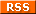feed! Your name Your email (optional) Validation Are you real? Please type 38413 backwards. Your comment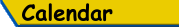Mon Tue Wed Thu Fri Sat Sun «   December 2021   » 1 2 3 4 5 6 7 8 9 10 11 12 13 14 15 16 17 18 19 20 21 22 23 24 25 26 27 28 29 30 31

(Felicity? Marte? Find out!)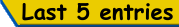List all b.log entries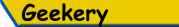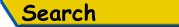Search Rick's b.log!
PS: Don't try to be clever.
It's a simple substring match.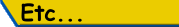Last read at 07:05 on 2023/09/23.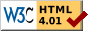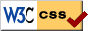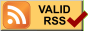© 2021 Rick Murray This web page is licenced for your personal, private, non-commercial use only. No automated processing by advertising systems is permitted. RIPA notice: No consent is given for interception of page transmission.

 Have you noticed the watermarks on pictures? Read the explanation.
Next entry - 2021/12/30